# MA.912.DP.1.1Export Print
Given a set of data, select an appropriate method to represent the data, depending on whether it is numerical or categorical data and on whether it is univariate or bivariate.

### Clarifications

Clarification 1: Instruction includes discussions regarding the strengths and weaknesses of each data display.

Clarification 2: Numerical univariate includes histograms, stem-and-leaf plots, box plots and line plots; numerical bivariate includes scatter plots and line graphs; categorical univariate includes bar charts, circle graphs, line plots, frequency tables and relative frequency tables; and categorical bivariate includes segmented bar charts, joint frequency tables and joint relative frequency tables.
Clarification 3: Instruction includes the use of appropriate units and labels and, where appropriate, using technology to create data displays.

General Information
Subject Area: Mathematics (B.E.S.T.)
Strand: Data Analysis and Probability
Status: State Board Approved

## Benchmark Instructional Guide

### Terms from the K-12 Glossary

• Categorical Data
• Numerical Data

### Vertical Alignment

Previous Benchmarks

Next Benchmarks

### Purpose and Instructional Strategies

In middle grades, students used box plots and histograms to display univariate numerical data; then bar charts, circle graphs and line plots to display univariate categorical data; and finally scatter plots and line graphs to display bivariate numerical data. In Algebra I, display univariate data and bivariate numerical data using graphical representations from middle grades and are introduced to bivariate categorical data, which they represent with frequency tables and segmented bar charts. Additionally, they must choose an appropriate display when considering each of the four varieties of data. In later courses, students will build upon this foundation as students consider a variety of data distributions in greater detail, including normal and Poisson distributions.
• While the benchmark states that students select an appropriate data display, instruction also includes cases where students must create the display.
• This benchmark is closely linked to MA.912.DP.1.2, where students interpret displayed data using key components of the display.
• Instruction includes student discussions (MTR.1.1) regarding the strengths and weaknesses of each data display, and includes the use of appropriate units and labels (MTR.4.1).
• Numerical univariate is data that consists of one numerical variable, and an important feature of the data is its numerical size or order. Examples include height, weight, age, salary, speed, number of pets, hours of study, etc. Displays include histograms, stem-and-leaf plots, box plots and line plots.
• Histograms
• Good for large sets of data.
• Shows the shape of the distribution to determine symmetry.
• Data is collected in suitably-sized numerical bins with equal ranges.
• Because of the bins, only approximate values of individual data points are displayed.
• Stem-and-Leaf Plots
• Good for small data sets.
• Shows the shape of a data set and each individual data value.
• Lists exact data values in a compact form.
• Box Plots
• Beneficial when large amounts of data are involved or compared. Used for descriptive data analysis.
• Shows multiple measures of variation and/or spread of data.
• Shows one measure of central tendency (median).
• Individual data points are not shown.
• Presents a 5-number summary of the data.
• Can indicate if a data set is skewed or not, but not the overall shape.
• Can be used to determine if potential outliers exist.
• Line Plots (Dot Plots)
• Used for small to moderate sized data sets in which the numerical values are discrete (often integers, or multiples of ½).
• Shows the shape of the distribution and the individual data points.
• Useful for highlighting clusters, gaps, and outliers.
• Numerical bivariate is data that involves two different numerical variables that have a possible relationship to each other. Displays include scatter plots and line graphs.
• Scatter Plots
• Good for large data sets, and for data sets in which it is not clear which variable, if any, should be considered the independent variable.
• Line Graphs
• Good for showing trends or cyclical patterns in small or medium-sized data sets in which there is an independent variable and a dependent variable. Often the values of the independent variable are chosen in advance by the person gathering the data. Examples of independent variables may be points in time or treatment amounts and examples of dependent variables might be total sales or average growth.
• Categorical univariate is non-numerical data of only one variable that can be categorized/grouped. Displays include bar charts, line plots, circle graphs, frequency tables and relative frequency tables.
• Bar Charts (Bar Graphs)
• Good for showing comparisons between categories or between different populations. A bar chart may show frequencies (counts) or relative frequencies (percentages) in each category.
• Circle Graphs
• Good for illustrating the percentage breakdown of items and visually representing a comparison. Not effective when there are too many categories. Shows how categories represent parts of a whole. A circle graph may show frequencies (counts) or relative frequencies (percentages) in each category.
• Frequency Tables and Relative Frequency Tables
• This is often the easiest way to display bivariate categorical data. The categories for one variable are listed in the header row of the table and the categories for the other variable are listed in the header column. The frequencies (counts) or relative frequencies (percentages) are listed in the cells for each of the indicated joint categories. Total counts or percentages for the rows may be listed in the final column of the table and total counts or percentages for the columns may be listed in the final row.
• Segmented Bar Charts
• Comparison of more than one categorical data sets.
• Good for showing the composition of the individual parts to the whole and making comparisons.
• Non-numerical data may consist of numbers if the categories are not primarily determined by the numerical size or order of the numbers.
• For example, the data may answer the question “What is your favorite real number?” and the categories could be “Integers,” “Rational numbers that are not integers” and “Irrational numbers.”
• Using the same real-world data (MTR.7.1), encourage students to create a variety of data displays appropriate for the data given (MTR.2.1). This makes the discussion of the similarities and differences of the displays more robust and allows students to visualize and justify their responses (MTR.3.1)
• This strategy might work best if you present the class with a set of data, group students and ask each group to create a different display using the same data.
• Each group can then present the strengths and weaknesses of their display as compared to the others (MTR.5.1)
• This should be repeated for each separate data category, see examples above.
• This benchmark references bar charts; however, other benchmarks and the glossary (Appendix C) reference bar graph, these terms are used interchangeably without difference.

### Common Misconceptions or Errors

• Students may not know how to label displays appropriately or how to choose appropriate units and scaling.
• For example, they may not know how to create or scale the number line for a line plot, they may confuse frequency and actual data values, or they may not understand that intervals for histograms should be done in equal increments.
• Students may not understand the meaning of quartiles in the box plot.
• Students may not know how to calculate the median with an even number of data values.
• Students may not accurately place data values in increasing order when there are many data points.
• Students may confuse bar charts (for categorical data) and histograms (for numerical data).
• Students may be confused when categorical data consists of numbers that have been categorized in ways that do not primarily reflect the numerical size or order of the numbers. In such cases, it will be helpful to have the student think about whether any of the measures of center (mean, median) or variability (quartiles, range) are meaningful for the data set. If they are, then the data can be considered numerical, because these measures are concerned with the numerical size and order of the data points. If not, then it can be considered categorical.

### Strategies to Support Tiered Instruction

• Teacher co-creates anchor charts that include appropriate units of measure.
• For example, time measurement units include seconds, minutes, hours, days, weeks, etc.
• Teacher provides numerical univariate, numerical bivariate, categorical univariate and categorical bivariate examples. Each example should include scaling to ensure that students have experience scaling for graphs and tables that are in each category.
• For example, employee ages for the company AdvertiseHere can be displayed using a box plot as shown.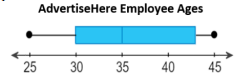• Teacher reviews the difference between histograms and bar graphs, creating an anchor chart with properties of a histogram for students to refer to.
• Teacher reinforces how scales are represented with specific endpoints. The endpoints they chose to use, or as defined in a problem, tell them if the point is included in the bin or not. Include notation of endpoints on anchor chart to display in the classroom.
• Teacher co-constructs vocabulary guide/anchor chart with students who need additional support understanding the vocabulary for measures of center and variation.
• Examples of guides and charts are shown below.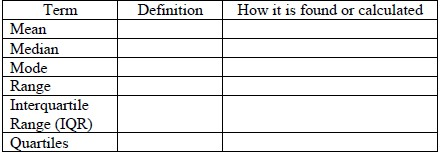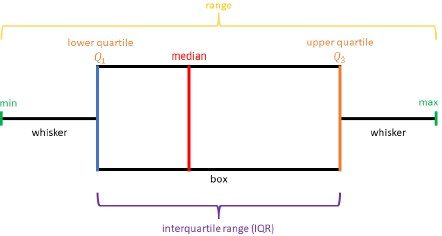• Teacher models ordering data sets in ascending order before finding a median, quartile or range.
• Teacher provides a chart to display calculating the median with an even and odd data set.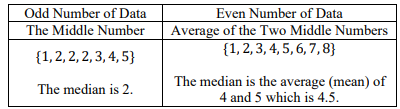• Instruction includes discussions about whether any of the measures of center (mean, median) or variability (quartiles, range) are meaningful for the data set. If they are, then the data can be considered numerical, because these measures are concerned with the numerical size and order of the data points. If not, then it can be considered categorical.

• The number of cars sold in a week at a large car dealership over a 20-week period is given below.
16 12 8 7 26 32 15 51 29 45 19 11 6 15 32 18 43 31 23 23
Which data display would you use to represent this data? Explain your reasoning.

• The following data set shows the change in the total amount of municipal waste generated in the United States during the 1990’s. Choose and create an appropriate data display to represent the information given.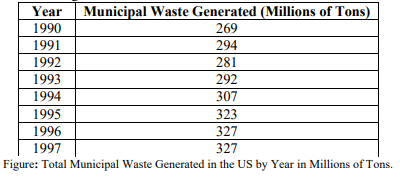Choose and create an appropriate data display to represent the information given.

• High school students in the United States were invited to complete an online survey in 2010. More than 1,000 students responded to this survey that included a question about a student?s favorite sport. 450 of the completed surveys were randomly selected. A breakdown of the data by gender was compiled from the 450 surveys.
• 100 students indicated their favorite sport was soccer. 49 of those students were females.
• 131 students selected lacrosse as their favorite sport. 71 of those students were males.
• 75 students selected basketball their favorite sport. 48 of those students were females.
• 26 students indicated football as their favorite sport. 25 of those students were males.
• 118 students indicated volleyball as their favorite sport. 70 of those students were females.
Choose and create an appropriate data display to represent the information given.

### Instructional Items

Instructional Item 1
• The following table shows the amount of tonnage of the most common types of electronic equipment discarded in the United States in 2005.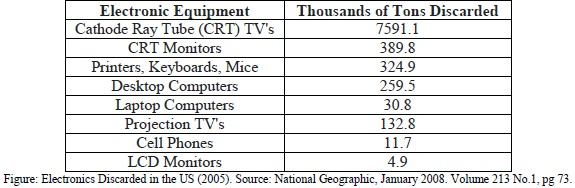Which data display would you use to represent this data? Explain your reasoning.

*The strategies, tasks and items included in the B1G-M are examples and should not be considered comprehensive.

## Related Courses

This benchmark is part of these courses.
1200310: Algebra 1 (Specifically in versions: 2014 - 2015, 2015 - 2022, 2022 and beyond (current))
1200320: Algebra 1 Honors (Specifically in versions: 2014 - 2015, 2015 - 2022, 2022 and beyond (current))
1200380: Algebra 1-B (Specifically in versions: 2014 - 2015, 2015 - 2022, 2022 and beyond (current))
1200400: Foundational Skills in Mathematics 9-12 (Specifically in versions: 2014 - 2015, 2015 - 2022, 2022 and beyond (current))
1210300: Probability and Statistics Honors (Specifically in versions: 2014 - 2015, 2015 - 2019, 2019 - 2022, 2022 and beyond (current))
7912070: Access Mathematics for Liberal Arts (Specifically in versions: 2014 - 2015, 2015 - 2018, 2018 - 2019, 2019 - 2022, 2022 - 2023, 2023 and beyond (current))
7912090: Access Algebra 1B (Specifically in versions: 2014 - 2015, 2015 - 2018, 2018 - 2019, 2019 - 2022, 2022 and beyond (current))
1200315: Algebra 1 for Credit Recovery (Specifically in versions: 2014 - 2015, 2015 - 2022, 2022 and beyond (current))
1200385: Algebra 1-B for Credit Recovery (Specifically in versions: 2014 - 2015, 2015 - 2022, 2022 and beyond (current))
7912075: Access Algebra 1 (Specifically in versions: 2014 - 2015, 2015 - 2018, 2018 - 2019, 2019 - 2022, 2022 and beyond (current))
1210305: Mathematics for College Statistics (Specifically in versions: 2022 and beyond (current))
1207350: Mathematics for College Liberal Arts (Specifically in versions: 2022 and beyond (current))

## Related Access Points

Alternate version of this benchmark for students with significant cognitive disabilities.
MA.912.DP.1.AP.1a: Given a set of data, select an appropriate table or graph to represent categorical data and whether it is univariate or bivariate.
MA.912.DP.1.AP.1b: Given a set of data, select an appropriate table or graph to represent numerical data and whether it is univariate or bivariate.

## Related Resources

Vetted resources educators can use to teach the concepts and skills in this benchmark.

## Formative Assessments

Winning Seasons:

Students are asked to construct a histogram corresponding to a given set of data.

Type: Formative Assessment

Trees in the Park:

Students are asked to construct a box plot corresponding to a given set of data.

Type: Formative Assessment

Flowering Trees:

Students are asked to determine whether each of two given dot plots are consistent with a given histogram.

Type: Formative Assessment

A Tomato Garden:

Students are asked to construct a dot plot corresponding to a given set of data.

Type: Formative Assessment

## Lesson Plans

Where Should I Go to College? :

Students will create and use data displays to determine which college is the right fit for him or her / for hypothetical students. They will justify the data displays they selected, present this information to classmates and write an essay justifying their choice.

Type: Lesson Plan

Choosing The Proper Chart For Your Data Set:

Students will use previously gathered data to create a spreadsheet, choose and create a graph/chart that best diplays the data, and explain their reasoning for choosing the graph/chart, in this lesson plan.

Type: Lesson Plan

Interpret Population Data with Graphs and Tables:

Students will use the calculated population totals to create graphs that help to visualize the totals for analyzing and representation. Census data is used as the data to provide information to analyze. Students will then use basic functions and formulas in spreadsheets to help analyze and represent the data.

Type: Lesson Plan

Scatter plots, spaghetti, and predicting the future:

Students will construct a scatter plot from given data. They will identify the correlation, sketch an approximate line of fit, and determine an equation for the line of fit. They will explain the meaning of the slope and y-intercept in the context of the data and use the line of fit to interpolate and extrapolate values.

Type: Lesson Plan

## Original Student Tutorials

Movies Part 2: What’s the Spread?:

Follow Jake along as he relates box plots with other plots and identifies possible outliers in real-world data from surveys of moviegoers' ages in part 2 in this interactive tutorial.

This is part 2 of 2-part series, click HERE to view part 1.

Type: Original Student Tutorial

Movies Part 1: What's the Spread?:

Follow Jake as he displays real-world data by creating box plots showing the 5 number summary and compares the spread of the data from surveys of the ages of moviegoers in part 1 of this interactive tutorial.

This is part 1 of 2-part series, click HERE to view part 2.

Type: Original Student Tutorial

## Perspectives Video: Professional/Enthusiast

Graphs Help Identify Cost-Effective Sea Turtle Conservation Strategies:

This marine biologist discusses her use of graphical representations to help determine the most cost-effective management strategies for sea turtle conservation.

Type: Perspectives Video: Professional/Enthusiast

## STEM Lessons - Model Eliciting Activity

Where Should I Go to College? :

Students will create and use data displays to determine which college is the right fit for him or her / for hypothetical students. They will justify the data displays they selected, present this information to classmates and write an essay justifying their choice.

## MFAS Formative Assessments

A Tomato Garden:

Students are asked to construct a dot plot corresponding to a given set of data.

Flowering Trees:

Students are asked to determine whether each of two given dot plots are consistent with a given histogram.

Trees in the Park:

Students are asked to construct a box plot corresponding to a given set of data.

Winning Seasons:

Students are asked to construct a histogram corresponding to a given set of data.

## Original Student Tutorials Mathematics - Grades 9-12

Movies Part 1: What's the Spread?:

Follow Jake as he displays real-world data by creating box plots showing the 5 number summary and compares the spread of the data from surveys of the ages of moviegoers in part 1 of this interactive tutorial.

This is part 1 of 2-part series, click HERE to view part 2.

Movies Part 2: What’s the Spread?:

Follow Jake along as he relates box plots with other plots and identifies possible outliers in real-world data from surveys of moviegoers' ages in part 2 in this interactive tutorial.

This is part 2 of 2-part series, click HERE to view part 1.

## Student Resources

Vetted resources students can use to learn the concepts and skills in this benchmark.

## Original Student Tutorials

Movies Part 2: What’s the Spread?:

Follow Jake along as he relates box plots with other plots and identifies possible outliers in real-world data from surveys of moviegoers' ages in part 2 in this interactive tutorial.

This is part 2 of 2-part series, click HERE to view part 1.

Type: Original Student Tutorial

Movies Part 1: What's the Spread?:

Follow Jake as he displays real-world data by creating box plots showing the 5 number summary and compares the spread of the data from surveys of the ages of moviegoers in part 1 of this interactive tutorial.

This is part 1 of 2-part series, click HERE to view part 2.

Type: Original Student Tutorial

## Lesson Plan

Interpret Population Data with Graphs and Tables:

Students will use the calculated population totals to create graphs that help to visualize the totals for analyzing and representation. Census data is used as the data to provide information to analyze. Students will then use basic functions and formulas in spreadsheets to help analyze and represent the data.

Type: Lesson Plan

## Parent Resources

Vetted resources caregivers can use to help students learn the concepts and skills in this benchmark.